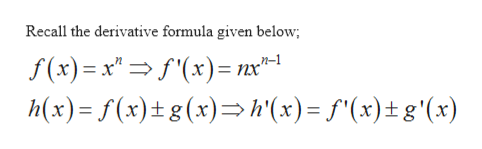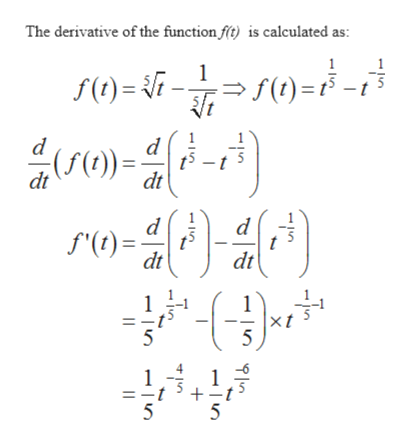# POiferentiate

Question

How would I differentiate the function?

check_circle

Step 1
Step 2help_outlineImage TranscriptioncloseRecall the derivative formula given below; f(x)= x x)= nx-1 h(x) f(x)t g(x)h'(x)= f"(x)tg'(x) fullscreen
Step 3help_outlineImage TranscriptioncloseThe derivative of the function f) is calculated as: 1 ) f(t)= t I0)=; dt d dt dt 1 xt 5 5 + 5 5 fullscreen

### Want to see the full answer?

See Solution

#### Want to see this answer and more?

Solutions are written by subject experts who are available 24/7. Questions are typically answered within 1 hour.*

See Solution
*Response times may vary by subject and question.
Tagged in

### Derivative